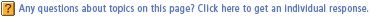MATHEMATICA LINK FOR EXCEL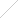Mathematica Link for ExcelFeatures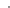ExcelLinkMathematicaLinkWhat's New in Version 3Who's It For?ExamplesUser's Manual and Reference GuideBuy Online# Features

Mathematica Link for Excel consists of two main components, the ExcelLink package and the MathematicaLink add-in. These components work together to provide full two-way connectivity between Mathematica and Excel.

### ExcelLink Features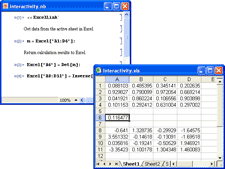A suite of Mathematica functions that allow you to: Read and write data to Excel ranges Display graphics, typeset equations and formatted output in Excel Create customized Excel import and export routines Develop Excel functions and macros in Mathematica A special clipboard window that allows you to easily copy and paste data between Mathematica and Excel Integrated documentation in the Mathematica Help Browser

### MathematicaLink Features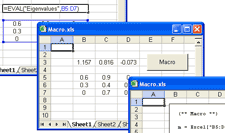A set of worksheet functions that allow you to use Mathematica functions in Excel formulas A Mathematica function wizard to help you learn about and enter Mathematica functions A Mathematica macros window that allows you to turn Mathematica code into Excel macros